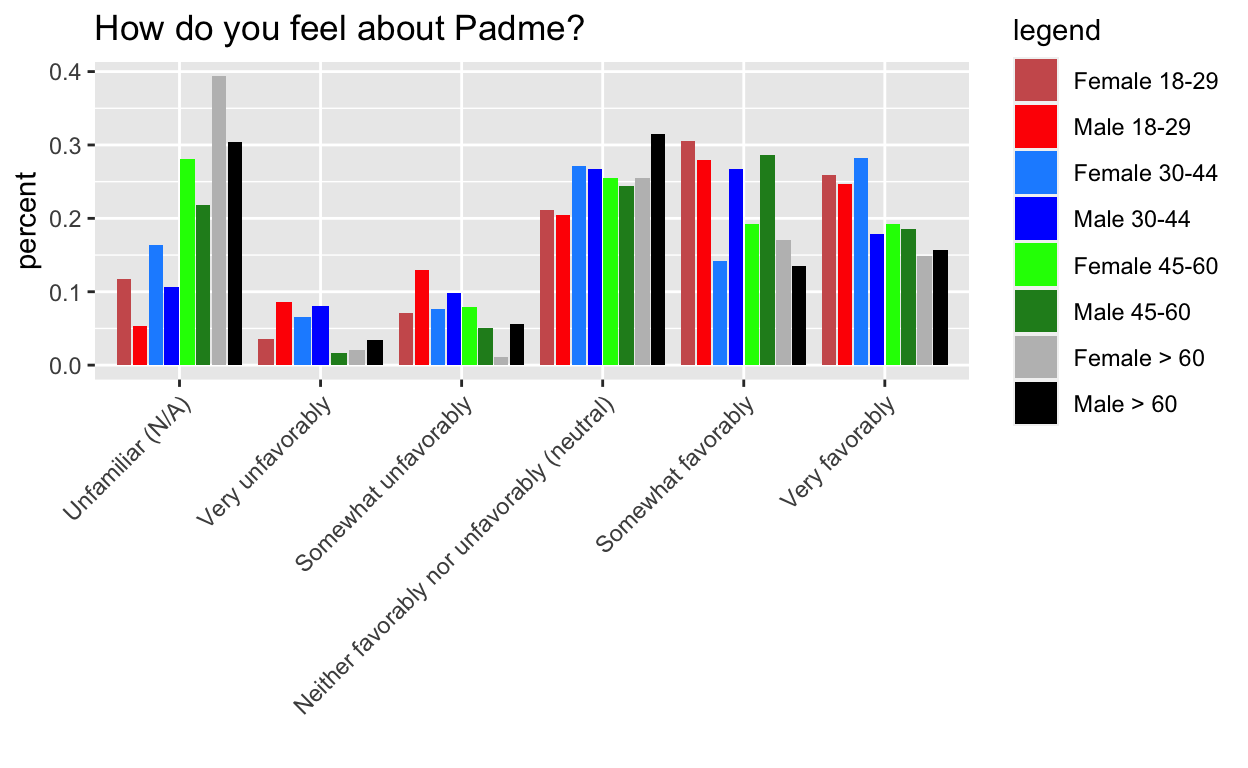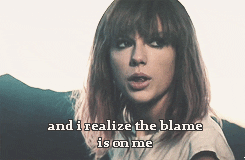# Troubles with Tibble

Sara Stoudt true
06-03-2018
``````require(readr)
require(dplyr)
require(ggplot2)
require(tibble)
require(data.table)
require(gridExtra)
``````

Note: I’m skipping over purrr until @kellieotto returns from her travels, so we can write our joint post.

This week’s post is on tibbles. This actually came at a perfect time since recently I’ve run into a few mysteries where I get unexpected errors or output after a data frame gets turned into a tibble at some point during my workflow (like when I use functions from the tidyverse). This is actually a good sign because it means I’m using the tidyverse more in my day to day work. So now to solve some tibble troubles.#### Mystery #1

This mystery comes from Week 7 of Tidy Tuesday, the dreaded barplots. I’m hiding the process code to streamline the post, but if you want to see it, go here. The gist of it, is that I want the order of the side by side bars to be consistent within category.

The key is to pre-arrange the data to follow the order that we want to plot it in.

g1 doesn’t work, but g2, g3, and g4 do.

Key: It turns out that the tibble format isn’t the issue per se, it is tibble’s lazy evaluation (and ggplot’s) that is the real issue. This is analogous to why you need `aes_string` instead of `aes` when you are passing in a variable name to ggplot in a custom made function. The rearranging doesn’t actually happen until wrapped in another function that evaulates it.

``````toPlot
``````
``````# A tibble: 47 x 7
# Groups:   Gender, Age 
Gender Age   V28                 count.x count.y percent genderAge
<fct>  <fct> <fct>                 <int>   <int>   <dbl> <fct>
1 Female > 60  Unfamiliar (N/A)         37      94  0.394  Female > …
2 Female > 60  Very unfavorably          2      94  0.0213 Female > …
3 Female > 60  Somewhat unfavorab…       1      94  0.0106 Female > …
4 Female > 60  Neither favorably …      24      94  0.255  Female > …
5 Female > 60  Somewhat favorably       16      94  0.170  Female > …
6 Female > 60  Very favorably           14      94  0.149  Female > …
7 Female 18-29 Unfamiliar (N/A)         10      85  0.118  Female 18…
8 Female 18-29 Very unfavorably          3      85  0.0353 Female 18…
9 Female 18-29 Somewhat unfavorab…       6      85  0.0706 Female 18…
10 Female 18-29 Neither favorably …      18      85  0.212  Female 18…
# … with 37 more rows``````
``````test <- toPlot %>% arrange(Age, V28)
is.tibble(test)
``````
`` TRUE``
``````test2 <- as.data.frame(toPlot %>% arrange(Age, V28))
test3 <- data.frame(toPlot %>% arrange(Age, V28))
test4 <- as.tibble(toPlot %>% arrange(Age, V28))
# test5=tibble(toPlot%>%arrange(Age,V28)) ## Error: Column `toPlot %>% arrange(Age, V28)` must be a 1d atomic vector or a list

g1 <- ggplot(test, aes(V28, y = percent, fill = genderAge)) +
geom_bar(stat = "identity", position = position_dodge2(preserve = "total")) +
theme(axis.text.x = element_text(angle = 45, hjust = 1)) +
xlab("") +
scale_fill_manual("legend", values = c("Female 18-29" = "indianred", "Male 18-29" = "red", "Female 30-44" = "dodgerblue", "Male 30-44" = "blue", "Female 45-60" = "green", "Male 45-60" = "forestgreen", "Female > 60" = "grey", "Male > 60" = "black"))

g2 <- ggplot(test2, aes(V28, y = percent, fill = genderAge)) +
geom_bar(stat = "identity", position = position_dodge2(preserve = "total")) +
theme(axis.text.x = element_text(angle = 45, hjust = 1)) +
xlab("") +
scale_fill_manual("legend", values = c("Female 18-29" = "indianred", "Male 18-29" = "red", "Female 30-44" = "dodgerblue", "Male 30-44" = "blue", "Female 45-60" = "green", "Male 45-60" = "forestgreen", "Female > 60" = "grey", "Male > 60" = "black"))

g3 <- ggplot(test3, aes(V28, y = percent, fill = genderAge)) +
geom_bar(stat = "identity", position = position_dodge2(preserve = "total")) +
theme(axis.text.x = element_text(angle = 45, hjust = 1)) +
xlab("") +
scale_fill_manual("legend", values = c("Female 18-29" = "indianred", "Male 18-29" = "red", "Female 30-44" = "dodgerblue", "Male 30-44" = "blue", "Female 45-60" = "green", "Male 45-60" = "forestgreen", "Female > 60" = "grey", "Male > 60" = "black"))

g4 <- ggplot(test4, aes(V28, y = percent, fill = genderAge)) +
geom_bar(stat = "identity", position = position_dodge2(preserve = "total")) +
theme(axis.text.x = element_text(angle = 45, hjust = 1)) +
xlab("") +
scale_fill_manual("legend", values = c("Female 18-29" = "indianred", "Male 18-29" = "red", "Female 30-44" = "dodgerblue", "Male 30-44" = "blue", "Female 45-60" = "green", "Male 45-60" = "forestgreen", "Female > 60" = "grey", "Male > 60" = "black"))

g1
````````````g2
````````````g3
````````````g4
``````#### Mystery #2

This mystery comes from a scenario at work. I had one dataset that had different states than another dataset (state was treated as a factor). I wanted to find new levels and replace them with a catch all “other” level. I started with a data frame, but I used `complete` from tidyr at some point, and unknowingly had switched to a tibble. Therefore, my subsetting procedure was not doing what I expected. Here is a simplified example:

``````state <- tibble(state = as.factor(c("AL", "AK", "AR", "AS")))
state2 <- tibble(state = as.factor(c("AL", "AK", "AR", "AS", "CA", "CO")))
``````

What I did:

``````state2[, 1] %in% levels(state[, 1])
``````
`` FALSE``
``````levels(state[, 1])
``````
``NULL``

What I should have did given that I’m working with tibbles:

``````test <- state2[] %in% levels(state[])
test
``````
``  TRUE  TRUE  TRUE  TRUE FALSE FALSE``

Key: subsetting tibbles by column requires the double brackets

What I did:

``````state2[, 1] <- as.character(state2[, 1])
state2
``````
``````# A tibble: 6 x 1
state
<chr>
1 c(2, 1, 3, 4, 5, 6)
2 c(2, 1, 3, 4, 5, 6)
3 c(2, 1, 3, 4, 5, 6)
4 c(2, 1, 3, 4, 5, 6)
5 c(2, 1, 3, 4, 5, 6)
6 c(2, 1, 3, 4, 5, 6)``````

What I should have did given that I’m working with tibbles:

Key: again this is a subsetting syntax issue

``````state2 <- tibble(state = as.factor(c("AL", "AK", "AR", "AS", "CA", "CO")))

state2[] <- as.character(state2[])
state2[test, 1] <- "other"
``````

Compare to behavior on a dataframe (this is the output I expected):

``````state <- data.frame(state = as.factor(c("AL", "AK", "AR", "AS")))
state2 <- data.frame(state = as.factor(c("AL", "AK", "AR", "AS", "CA", "CO")))

state2[, 1] %in% levels(state[, 1])
``````
``  TRUE  TRUE  TRUE  TRUE FALSE FALSE``
``````levels(state[, 1])
``````
`` "AK" "AL" "AR" "AS"``
``````state2[, 1] <- as.character(state2[, 1])
state2
``````
``````  state
1    AL
2    AK
3    AR
4    AS
5    CA
6    CO``````
``````state2[test, 1] <- "other"
state2
``````
``````  state
1 other
2 other
3 other
4 other
5    CA
6    CO``````
``````state2
``````
``````  state
1 other
2 other
3 other
4 other
5    CA
6    CO``````

#### Mystery #3

This mystery comes from my readr post where I wanted to remove rows that had NAs in certain columns and a certain number of characters in another example. Here is a simplified example:

``````tb <- tibble(x = c(NA, NA, 3), y = c(NA, 2, 1), z = c("A", "BC", "D"))
tb[, 1] ## tibble
``````
``````# A tibble: 3 x 1
x
<dbl>
1    NA
2    NA
3     3``````
``````tb[] ## vector
``````
`` NA NA  3``

The difference in subsetting syntax is a reoccurring issue. I use the syntax expecting my data to be a dataframe, but I forgot that tidyverse functions switch to tibbles.

``````tb[-which(is.na(tb[, 2]) & is.na(tb[, 1]) & nchar(tb[, 3]) <= 1), ]
``````
``````# A tibble: 0 x 3
# … with 3 variables: x <dbl>, y <dbl>, z <chr>``````

Weird! I expected the output to remove the first row. What’s going on?

``````is.na(tb[, 2])
``````
``````         y
[1,]  TRUE
[2,] FALSE
[3,] FALSE``````
``````is.na(tb[, 1])
``````
``````         x
[1,]  TRUE
[2,]  TRUE
[3,] FALSE``````

These are fine.

``````nchar(tb[, 3]) <= 1
``````
``````    z
FALSE ``````
``````nchar(tb[, 3])
``````
`````` z
17 ``````

Ah, here is the culprit! Switching to tibble subsetting syntax…

``````nchar(tb[]) <= 1
``````
``  TRUE FALSE  TRUE``

Much better.

Original:

``````is.na(tb[, 2]) & is.na(tb[, 1]) & nchar(tb[, 3]) <= 1
``````
``````         y
[1,] FALSE
[2,] FALSE
[3,] FALSE``````

Fix:

``````is.na(tb[, 2]) & is.na(tb[, 1]) & nchar(tb[]) <= 1
``````
``````         y
[1,]  TRUE
[2,] FALSE
[3,] FALSE``````
``````tb[-which(is.na(tb[, 2]) & is.na(tb[, 1]) & nchar(tb[]) <= 1), ]
``````
``````# A tibble: 2 x 3
x     y z
<dbl> <dbl> <chr>
1    NA     2 BC
2     3     1 D    ``````

Compare to behavior on a dataframe:

``````tb <- tibble(x = c(NA, NA, 3), y = c(NA, 2, 1), z = c("A", "BC", "D"))
tb2 <- as.data.frame(tb)

tb2[-which(is.na(tb2[, 2]) & is.na(tb2[, 1]) & nchar(tb2[, 3]) <= 1), ]
``````
``````   x y  z
2 NA 2 BC
3  3 1  D``````

This is the output I expected.

``````is.na(tb2[, 2])
``````
``  TRUE FALSE FALSE``
``````is.na(tb2[, 1])
``````
``  TRUE  TRUE FALSE``
``````nchar(tb2[, 3]) <= 1
``````
``  TRUE FALSE  TRUE``

The double bracket subsetting also works:

``````is.na(tb2[])
``````
``  TRUE FALSE FALSE``
``````is.na(tb2[])
``````
``  TRUE  TRUE FALSE``
``````nchar(tb2[]) <= 1
``````
``  TRUE FALSE  TRUE``
``````is.na(tb2[, 2]) & is.na(tb2[, 1]) & nchar(tb2[, 3]) <= 1
``````
``  TRUE FALSE FALSE``
``````is.na(tb2[]) & is.na(tb2[]) & nchar(tb2[]) <= 1
``````
``  TRUE FALSE FALSE``

Take-Away: The unexpected behavior that led to most of these mysteries turned out to be because I was using the wrong subsetting syntax.Since the double bracket subsetting works for dataframes and tibbles, I should transition to using this syntax so that I am not surprised by output when a tibble gets thrown into the mix.

Resources (these helped me solve my mysteries):